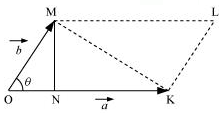## Pages

### System of particles and Rotational motion NCERT Solutions Class 11 Physics - Solved Exercise Question 7.4

Question 7.4:
Show that the area of the triangle contained between the vectors a and b is one half of the magnitude of a × b.
Solution:
Consider two vectors OK = a and OM = b, inclined at an angle θ, as shown in the following figure.In ΔOMN, we can write the relation:
Sin θ = MN / OM  =  MN / |b|
MN = |b| Sin θ
|a X a| = |a| |b| Sin θ
= OK X MN X 2 / 2
= 2 × Area of ΔOMK
∴ Area of ΔOMK = (1/2) |a X b|